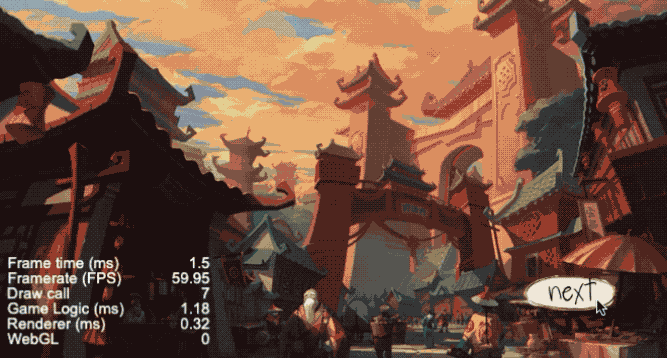# 效果演示# 实现思路

``````void main () {
vec4 color = vec4(1, 1, 1, 1);
color *= texture(texture, v_uv0);
color *= v_color;

#if USE_TRAMSFORM
#endif

gl_FragColor = color;
}
``````

``````isTransforming: boolean = false;
bgTramsform() {
if (this.isTransforming) return;
this.isTransforming = true;

let time = 0.0;
let node = this.switchNodeList;
let material = node.getComponent(cc.Sprite).getMaterial(0);
material.setProperty('u_time', time);
material.define('USE_TRAMSFORM', true, 0, true);

let timer = setInterval(() => {
time += 0.03;
material.setProperty('u_time', time);
this.switchNodeList.shift();
this.switchNodeList.push(node);
this.switchNodeList.forEach((node, idx) => node.zIndex = this.switchNodeList.length - idx)
material.define('USE_TRAMSFORM', false, 0, true);
this.isTransforming = false;
timer && clearInterval(timer);
}
}, 30);
}
``````## 效果预览